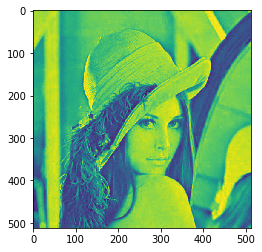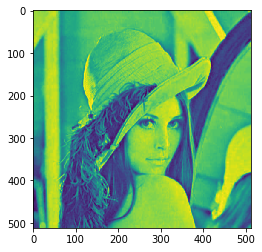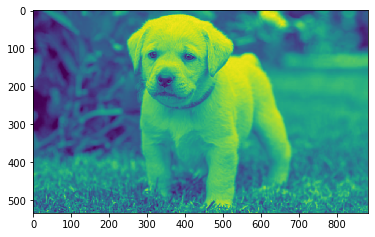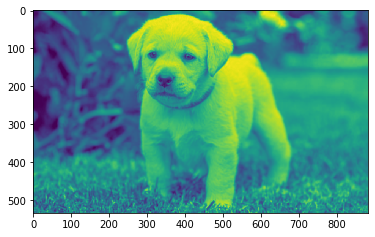GFG App
Open AppBrowser
Continue

# Mahotas – Mean filter

In this article we will see how we can apply mean filter to the image in mahotas.Average (or mean) filtering is a method of ‘smoothing’ images by reducing the amount of intensity variation between neighbouring pixels. The average filter works by moving through the image pixel by pixel, replacing each value with the average value of neighbouring pixels, including itself.
In this tutorial we will use “lena” image, below is the command to load it.

`mahotas.demos.load('lena')`

Below is the lena imageIn order to do this we will use mahotas.mean_filter method
Syntax : mahotas.mean_filter(img, n)
Argument : It takes image object and neighbor pixel as argument
Return : It returns image object

Note : Input image should be filtered or should be loaded as grey

In order to filter the image we will take the image object which is numpy.ndarray and filter it with the help of indexing, below is the command to do this

`image = image[:, :, 0]`

Below is the implementation

## Python3

 `# importing required libraries` `import` `mahotas` `import` `mahotas.demos` `from` `pylab ``import` `gray, imshow, show` `import` `numpy as np` `import` `matplotlib.pyplot as plt` `  `  `# loading image` `img ``=` `mahotas.demos.load(``'lena'``)` `  `  `# filtering image` `img ``=` `img.``max``(``2``)`   `print``(``"Image"``)` `  `  `# showing image` `imshow(img)` `show()`   `# applying mean filter` `new_img ``=` `mahotas.mean_filter(img, n)` ` `    `# showing image` `print``(``"Mean Filter"``)` `imshow(new_img)` `show()`

Output :

`Image``Mean Filter`Another example

## Python3

 `# importing required libraries` `import` `mahotas` `import` `numpy as np` `from` `pylab ``import` `gray, imshow, show` `import` `os` `import` `matplotlib.pyplot as plt` ` `  `# loading image` `img ``=` `mahotas.imread(``'dog_image.png'``)`     `# filtering image` `img ``=` `img[:, :, ``0``]` `  `  `print``(``"Image"``)` `  `  `# showing image` `imshow(img)` `show()`   `# applying mean filter` `new_img ``=` `mahotas.mean_filter(img, n)` ` `    `# showing image` `print``(``"Mean Filter"``)` `imshow(new_img)` `show()`

Output :

`Image``Mean Filter`My Personal Notes arrow_drop_up